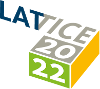#The 39th International Symposium on Lattice Field Theory (Lattice 2022)

Aug 8 – 13, 2022
Hörsaalzentrum Poppelsdorf
Europe/Berlin timezone

## Lattice calculation of leading isospin breaking effects in $\Gamma(K_{\ell 2})/\Gamma(\pi_{\ell 2})$ with close-to-physical chiral fermions

Aug 10, 2022, 2:00 PM
20m
CP1-HSZ/1st-1.002 - HS6 (CP1-HSZ)

### CP1-HSZ/1st-1.002 - HS6

#### CP1-HSZ

50
Oral Presentation Weak Decays and Matrix Elements

### Speaker

Matteo Di Carlo (University of Edinburgh)

### Description

In this talk we present the first RBC-UKQCD lattice calculation of the leading isospin-breaking corrections to the ratio of leptonic decay rates of kaons and pions into muon and neutrino, $\Gamma(K_{\ell 2})/\Gamma(\pi_{\ell 2})$. This computation is performed using domain wall fermions with close-to-physical (light and strange) quark masses. The QED effects are implemented using a perturbative approach and infrared divergences are regulated according to the QED$_\mathrm{L}$ prescription. We describe the strategy to extract the relevant hadronic matrix elements from Euclidean correlation functions and we discuss the important role of finite volume effects in this calculation.

### Primary author

Matteo Di Carlo (University of Edinburgh)